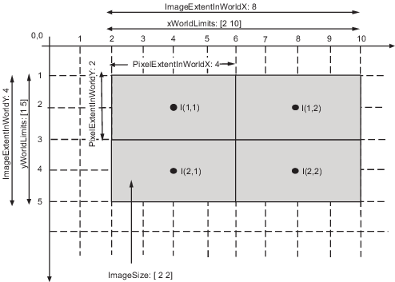## Define World Coordinate System of Image

The world coordinate system is a continuous spatial coordinate system that specifies the location in an image independently of the pixel indices of the image. For more information about coordinate systems in Image Processing Toolbox™, see Image Coordinate Systems.

### Define Spatial Referencing Objects

To specify a world coordinate system for an image, you can use spatial referencing objects. Spatial referencing objects define the location of the image in a world coordinate system and specify how the extents of the image relate to intrinsic and world limits. You can use these objects to specify nonsquare pixel dimensions by specifying a different image resolution in each dimension. Spatial referencing objects also enable you to convert between coordinate systems.

Image Processing Toolbox uses includes two spatial referencing objects, `imref2d` and `imref3d`. The table describes the properties of the 2-D spatial referencing object, `imref2d`. The 3-D spatial referencing object, `imref3d`, includes these properties as well as corresponding properties for the Z dimension.

PropertyDescription
`XWorldLimits`Upper and lower bounds along the X dimension in world coordinates (nondefault spatial coordinates)
`YWorldLimits`Upper and lower bounds along the Y dimension in world coordinates (nondefault spatial coordinates)
`ImageSize`Size of the image, returned by the `size` function.
`PixelExtentInWorldX`Size of pixel along the X dimension
`PixelExtentInWorldY`Size of pixel along the Y dimension
`ImageExtentInWorldX`Size of image along the X dimension
`ImageExtentInWorldY`Size of image along the Y dimension
`XIntrinsicLimits`Upper and lower bounds along X dimension in intrinsic coordinates (default spatial coordinates)
`YIntrinsicLimits`Upper and lower bounds along Y dimension in intrinsic coordinates (default spatial coordinates).

To illustrate spatial referencing, this sample code creates a spatial referencing object associated with a 2-by-2 image. The code specifies the pixel extent in the horizontal and vertical directions as 4 units/pixel and 2 units/pixel, respectively. The object calculates the world limits, image extent in world coordinates, and image extent in intrinsic coordinates.

`R = imref2d([2 2],4,2)`
```R = imref2d with properties: XWorldLimits: [2 10] YWorldLimits: [1 5] ImageSize: [2 2] PixelExtentInWorldX: 4 PixelExtentInWorldY: 2 ImageExtentInWorldX: 8 ImageExtentInWorldY: 4 XIntrinsicLimits: [0.5000 2.5000] YIntrinsicLimits: [0.5000 2.5000]```

The figure illustrates how these properties map to elements of the image.### Specify Minimum and Maximum Image Extent

`Image` objects (such as obtained when displaying an image using `imshow`) define the world extent using the `XData` and `YData` properties. Each property is a two-element vector that specifies the center coordinate of the outermost pixels in that dimension. For more information, see Image Properties.

By default, the intrinsic coordinates, world coordinates, and MATLAB® axes coordinates of an image coincide. For an image `A`, the default value of `XData` is `[1 size(A,2)]` and the default value of `YData` is `[1 size(A,1)]`. For example, if `A` is a 100 row by 200 column image, the default `XData` is [1 200] and the default `YData` is [1 100].

To define a nondefault world coordinate system for an image, specify the image `XData` and `YData` properties with the range of coordinates spanned by the image in each dimension. When you do this, the MATLAB axes coordinates become identical to the world coordinates and no longer coincide with the intrinsic coordinates. For an example, see Shift X- and Y-Coordinate Range of Displayed Image.

Note that the values in `XData` and `YData` are actually the coordinates for the center point of the boundary pixels, not the outermost edge of the boundary pixels. Therefore, the actual coordinate range spanned by the image is slightly larger. For instance, if `XData` is [1 200] and the image is 200 pixels wide, as for the intrinsic coordinate system, then each pixel is one unit wide and the interval in `X` spanned by the image is [0.5 200.5]. Similarly, if `XData` is [1 200] and the image is 50 pixels wide, as for a nondefault world coordinate system, then each pixel is four units wide and the interval in `X` spanned by the image is [–1 202].

You can set `XData` or `YData` such that the x-axis or y-axis is reversed. You would do this by placing the larger value first. For example, set the `XData` to [200 1].